# What does this recursive function do

## Recursion explained

### What is recursion?

Recursion is a programming concept in which a function does only a small part of the work, shrinking a problem a bit, and then calling itself to solve the rest of the problem.

This continues until the problem is reduced to a very simple case.

### An example

A classic example of explaining recursion is the so-called factorial function.

It is defined as follows:

n! = n * (n-1) * ... * 2 * 1

That is, the factorial of a number is the product of all integers less than or equal to the number itself.

The above definition is not very elegant: although it is obvious what is meant, strictly speaking it does not provide any meaningful values ​​because one appears in the definition.

The more elegant definition goes like this:

n! = 1 if n = 1 n! = n * (n-1)! otherwise

Note that in the definition of the faculty, the faculty itself appears, nevertheless it is meaningfully defined.

This form of definition is based very closely on recursive programming. Programmed in C, this function looks like this:

int faculty (int n) {if (n == 1) {return1; } else {return n * faculty (n-1); }}

What happens now when you call?

In the first call, the condition is certainly not met, so the second branch is called and returned.

But the value for is not known, so the function has to be calculated again, this time with the argument.

The call to does not return a pure number either, but and is finite.

So the following was calculated:

faculty (3) = 3 * faculty (2) = 3 * 2 * faculty (1) = 3 * 2 * 1 = 6

### Why all this?

Anyone who has seen this example is probably wondering what the recursion is supposed to do. After all, a very simple, iterative (i.e. non-recursive) program does the same thing:

int faculty (int n) {int p = 1; while (n> 1) {p = p * n; n--; } return p; }

And it's faster too.

However, recursion has the advantage that it naturally breaks down larger problems into smaller ones, making it sometimes much easier to tackle.

Would you like an example? Take the "Towers of Hanoi".

This is an old game where you have three posts with rings of different sizes on them. The object of the game is to move the tower to one of the other posts without ever moving two rings at once or placing a larger one on top of a smaller ring.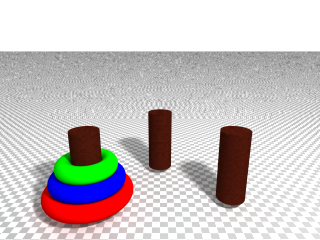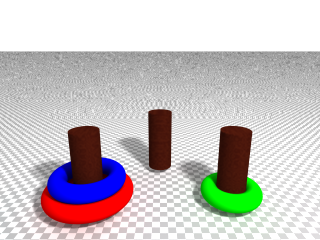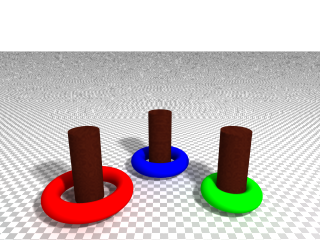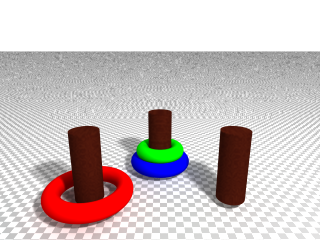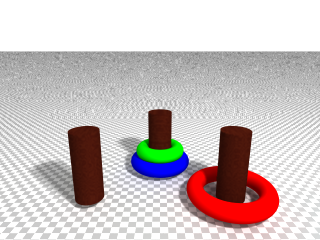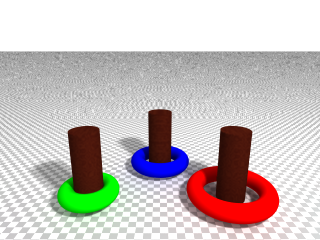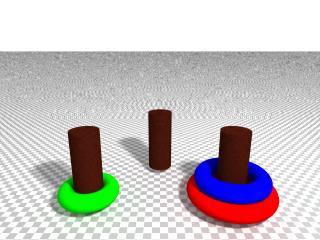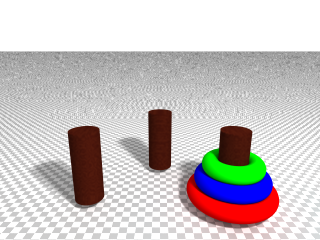The solution strategy can be described as follows: if you only want to move one ring, you can just do it. If you want to move several rings, you first move all but the bottom one onto the intermediate pile, move the last ring and then move the rest of the pile to its end position over the moved ring.

Or as a C program:

void move (int coin, char start, char end) {printf ("Moving coin% d from '% c' to '% c' \ n", start, start, end); } void hanoi (int coin, char start, char end, char third) {if (coin == 1) {move (1, start, end); } else {hanoi (coin - 1, start, third, end); move (coin, start, end); hanoi (coin - 1, third, end, start); }} int main (int argc, char ** argv) {hanoi_move (3, 'A', 'B', 'C'); return0; }

It's hard to believe that this simple code is supposed to solve the problem, but it really is.

To illustrate this, you can "manually" consider the order in which the calls are made.

To save space, I replace all calls to sub-functions in each level, although they are seen one after the other in the program (and not at the same time)

0th level: hanoi (3, 'A', 'B', 'C'); 1st level: hanoi (2, 'A', 'C', 'B'); move ('A', 'C'); hanoi (2, 'C', 'B', 'A'); 2nd level: hanoi (1, 'A', 'B', 'C'); move ('A', 'C'); hanoi (1, 'C', 'B', 'A'); move ('A', 'C'); hanoi (1, 'C', 'A', 'B'); move ('C', 'B'); hanoi (1, 'A', 'B', 'C'); 3rd level: move ('A', 'B'); move ('A', 'C'); move ('C', 'B'); move ('A', 'C'); move ('C', 'A'); move ('C', 'B'); move ('A', 'B');

So first a ring is moved from to. It took the program three function calls to find out.

These steps are typical for recursive functions:

• A termination condition that ensures that there is no endless loop
• A small part of the problem is solved in the function itself, the rest is solved recursively by itself
• If necessary, the two solutions are combined.

### Another example: Merge Sort

The towers of Hanoi are a more academic example. Often in the programmer's wilderness one encounters the problem of having to sort a list. A popular and quick method is Merge Sort.

Merge Sort works like this:

• If the input list contains one or no item, it is sorted
• Split the list in the middle. Sort the two halves recursively
• Combine the two sorted lists into a common sorted list (according to the zipper principle).

And implemented again in C:

#include void mergesort (int * const list, constint length) {if (length <= 1) {return; } int half = length / 2; / * sort the left half * / mergesort (list, half); / * sort the right half * / mergesort (list + half, length - half); / * join the sorted half * / int left_p = 0; int right_p = half; int new_values ​​[length]; for (int i = 0; i